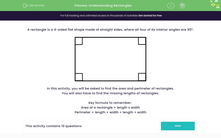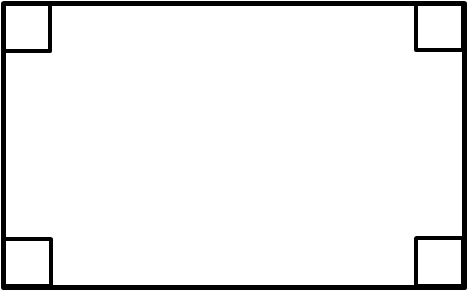# Understanding Rectangles

In this worksheet, students will be asked to find missing lengths of rectangles from their given area. Students will also be asked to calculate perimeter once a missing length has been found.Key stage:  KS 2

Curriculum topic:   Geometry: Properties of Shapes

Curriculum subtopic:   Understand Rectangles

Popular topics:   Shape worksheets

Difficulty level:#### Worksheet Overview

A rectangle is a 4-sided flat shape made of straight sides, where all four of its interior angles are 90°.In this activity, you will be asked to find the area and perimeter of rectangles.

You will also have to find the missing lengths of rectangles.

Key formula to remember:

Area of a rectangle = length x width

Perimeter = length + width + length + width

### What is EdPlace?

We're your National Curriculum aligned online education content provider helping each child succeed in English, maths and science from year 1 to GCSE. With an EdPlace account you’ll be able to track and measure progress, helping each child achieve their best. We build confidence and attainment by personalising each child’s learning at a level that suits them.

Get started